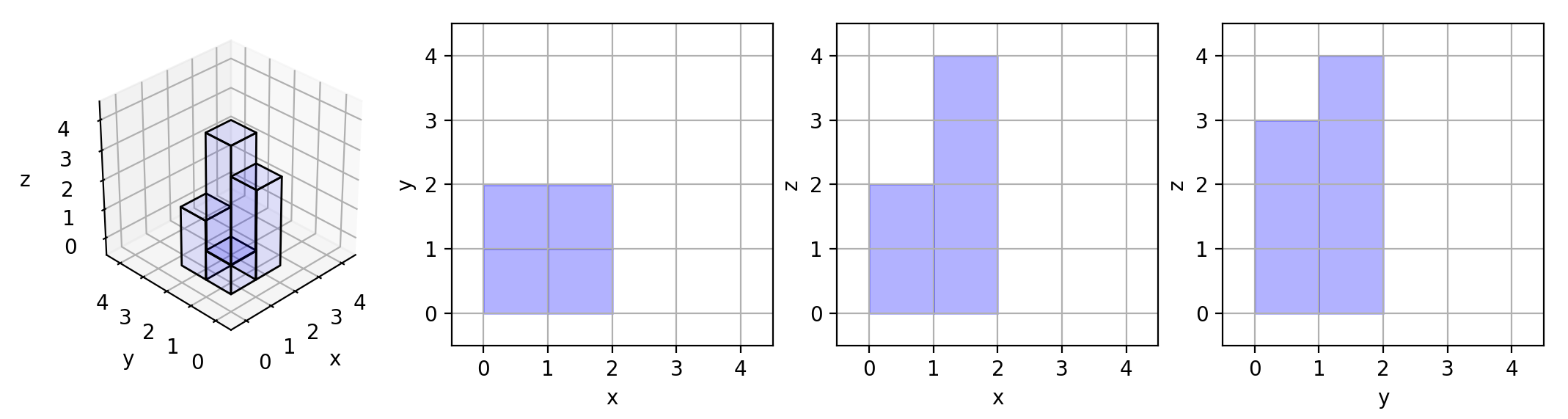Formatted question description: https://leetcode.ca/all/883.html

# 883. Projection Area of 3D Shapes (Easy)

On a N * N grid, we place some 1 * 1 * 1 cubes that are axis-aligned with the x, y, and z axes.

Each value v = grid[i][j] represents a tower of v cubes placed on top of grid cell (i, j).

Now we view the projection of these cubes onto the xy, yz, and zx planes.

A projection is like a shadow, that maps our 3 dimensional figure to a 2 dimensional plane.

Here, we are viewing the "shadow" when looking at the cubes from the top, the front, and the side.

Return the total area of all three projections.

Example 1:

Input: []
Output: 5


Example 2:

Input: [[1,2],[3,4]]
Output: 17
Explanation:
Here are the three projections ("shadows") of the shape made with each axis-aligned plane.Example 3:

Input: [[1,0],[0,2]]
Output: 8


Example 4:

Input: [[1,1,1],[1,0,1],[1,1,1]]
Output: 14


Example 5:

Input: [[2,2,2],[2,1,2],[2,2,2]]
Output: 21


Note:

• 1 <= grid.length = grid.length <= 50
• 0 <= grid[i][j] <= 50

Related Topics:
Math

## Solution 1.

// OJ: https://leetcode.com/problems/projection-area-of-3d-shapes/
// Time: O(MN)
// Space: O(1)
class Solution {
public:
int projectionArea(vector<vector<int>>& grid) {
int cnt = 0;
for (int N = grid.size(), i = 0; i < N; ++i) {
int max1 = 0, max2 = 0;
for (int j = 0; j < N; ++j) {
if (grid[i][j]) ++cnt;
max1 = max(max1, grid[i][j]);
max2 = max(max2, grid[j][i]);
}
cnt += max1 + max2;
}
return cnt;
}
};


Java

• class Solution {
public int projectionArea(int[][] grid) {
int areaTop = 0, areaFront = 0, areaSide = 0;
int rows = grid.length, columns = grid.length;
int[] maxEachRow = new int[rows];
int[] maxEachColumn = new int[columns];
for (int i = 0; i < rows; i++) {
for (int j = 0; j < columns; j++) {
int cell = grid[i][j];
if (cell != 0)
areaTop++;
maxEachRow[i] = Math.max(maxEachRow[i], cell);
maxEachColumn[j] = Math.max(maxEachColumn[j], cell);
}
}
for (int num : maxEachRow)
areaSide += num;
for (int num : maxEachColumn)
areaFront += num;
return areaTop + areaFront + areaSide;
}
}

• // OJ: https://leetcode.com/problems/projection-area-of-3d-shapes/
// Time: O(MN)
// Space: O(1)
class Solution {
public:
int projectionArea(vector<vector<int>>& grid) {
int cnt = 0;
for (int N = grid.size(), i = 0; i < N; ++i) {
int max1 = 0, max2 = 0;
for (int j = 0; j < N; ++j) {
if (grid[i][j]) ++cnt;
max1 = max(max1, grid[i][j]);
max2 = max(max2, grid[j][i]);
}
cnt += max1 + max2;
}
return cnt;
}
};

• class Solution(object):
def projectionArea(self, grid):
"""
:type grid: List[List[int]]
:rtype: int
"""
top, front, side = 0, 0, 0
n = len(grid)
for i in range(n):
x, y = 0, 0
for j in range(n):
if grid[i][j] != 0:
top += 1
x = max(x, grid[i][j])
y = max(y, grid[j][i])
front += x
side += y